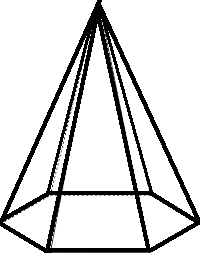## Pyramid Calculator

Calculate the various properties of Regular Hexagonal Pyramid, Regular Pentagonal Pyramid, Regular Square Pyramid and Regular Triangular Pyramid for given values.Regular Triangular Pyramid Area of Base :[ ½as ] Surface Area of Pyramid :[ ½as + (3/2)sl ] Volume of Pyramid :[ (1/6)abh ] Enter the Apothem Length = Enter the Side Length          = Enter the Slant height          = Enter the Height                  = Area of Base = Surface Area of Pyramid = Volume of Pyramid =Regular Square Pyramid Area of Base :[ s² ] Surface Area of Pyramid :[ s² + 2sl ] Volume of Pyramid :[ (1/3)b²h ] Enter the Side Length = Enter the Slant height = Enter the Height         = Area of Base = Surface Area of Pyramid = Volume of Pyramid =Regular Pentagonal Pyramid Area of Base :[ (5/2)as ] Surface Area of Pyramid :[ (5/2)as + (5/2)sl ] Volume of Pyramid :[ (5/6)abh ] Enter Apothem Length = Enter the Side Length = Enter the Slant height = Enter the Height         = Area of Base = Surface Area of Pyramid = Volume of Pyramid =Regular Hexagonal Pyramid Area of Base :[ (6/2)as ] Surface Area of Pyramid :[ 3as + 3sl ] Volume of Pyramid :[ abh ] Enter Apothem Length = Enter the Side Length = Enter the Slant height = Enter the Height         = Area of Base = Surface Area of Pyramid = Volume of Pyramid =

The pyramids are three-dimensional (3-D) shape whose base is a polygon and each corner of the polygon is attached to a singular apex that gives distinctive shape to the pyramids. The apex and each base edge form a triangle.
 Types of Pyramids Base Shape Triangular pyramid TriangleSquare pyramid SquarePentagonal pyramid PentagonHexagonal Pyramid HexagonalRight pyramid pyramid has apex above the center of its base